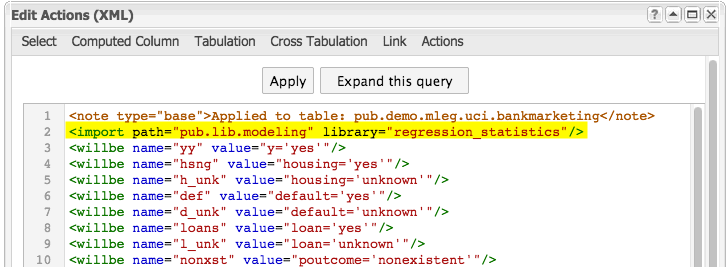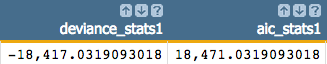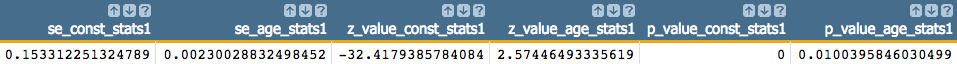# Extract logistic regression fit statistics

For a particular model, you can extract various fit statistics such as deviance, AIC, p-values, z-values, and standard errors. These statistics can be calculated using a 1010data-supplied library and inserting the associated block code within your query.

You must have already generated a model using `g_logreg(G;S;Y;XX;Z)`.

To extract the logistic regression fit statistics:

1. Import the `regression_statistics` library, which can be found in pub.lib.modeling.

This library contains the block `logreg_stats`, which we will use to calculate the fit statistics.

``<import path="pub.lib.modeling" library="regression_statistics"/>``
Note: It is best practice to put the `<import>` operation at the top of your Macro Language code; however, the only requirement is that it appears prior to any `<insert>` operation that references block code within the specified library.2. Insert the `logreg_stats` block at the end of your query.
The block `logreg_stats` takes a number of parameters: `model`, `target`, `arg_list`, `get`, and `ns`. We will call this block and specify the parameters using the `<insert>` operation.
Note: For more information on the parameters or the actual block code, see Block code: logreg_stats.
``````<insert block="logreg_stats" model="model" target="yy"
arg_list="age duration previous empvarrate hsng h_unk def d_unk
loans l_unk nonxst succ blue tech j_unk svcs mgmt ret entr self
maid unemp stud marr sgl m_unk" get="all" ns="_stats1"/>``````

A number of new columns containing the various fit statistics (as well as some intermediary columns that are used in the calculation of the fit statistics) will be added to the table.

For example, the following columns show deviance and AIC:The columns below show the standard error, z-values, and p-values for both the intercept (indicated by `const` in the column names) and the `age` variable: